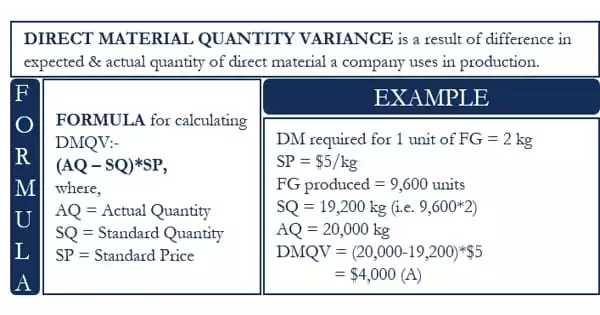Accounting

# Concept of Material Usage or Quantity Variance (MUV)The discrepancy between the actual amount of materials used in the manufacturing process and the amount planned to be used is referred to as a material quantity variation. The measurement is used to determine how efficient a manufacturing process is at converting raw materials into finished goods. Materials quantity variation is one of the cost accounting measures that firms examine in order to determine manufacturing efficiency. Keeping an eye on variations aids manufacturers in identifying and resolving issues as they arise. It also improves the precision of future production budgets.

The discrepancy between the standard quantities of material specified and the actual quantity of output and real quantities of material utilized is referred to as material usage variation or quantity variance. The material usage variance can be calculated by multiplying the difference between the actual quantity and the standard quantity by the standard price. As such the formula for the calculation of material usage variance is as follows:

Material Usage Variance (MUV) = SP x (RSQ – AQ)

Or, MUV = MMV + MYV

Where,

• SP = Standard price
• RSQ = Revised standard quantity
• AQ = Actual Quantity
• MMV = Material mix variance
• MYV = Material yield variance

If the actual quantity is less than the standard quantity, there will be a favorable variance and if the actual quantity is more than the standard quantity, there will be an unfavorable variance.

Calculation of Material Usage Variance

Illustration

• Standard quantity of material Q for 500 units of output is fixed as 800 kg.
• Standard price per kg. of material Q is estimated to be \$ 5
• Actual quantity of material Q was 800 kg.
• Actual price of material was \$ 4.5 per kg.
• Actual output was 400 units.

Solution,

Since the standard outputs and actual outputs differ, the standard quantity should be revised.

Revised standard quantity (RSQ) = (SQ/SO) x AO =800/500 x 400 = 640 units.

Now, Material usage variance (MUV) = SO x (RSQ – AQ) = 5 x (640-800) = \$ 800(U)

Since the actual quantity is more than standard quantity, the resulting variance is unfavorable variance.

Explanation

Because it is based on a standard unit quantity that may not be even close to real usage, the material quantity variance can produce unexpected outcomes. The engineering department normally determines the material quantity, which is based on the amount of material that should theoretically be utilized in the production process, as well as a provision for an acceptable amount of scrap. If the standard is overly lenient, there will be a long series of favorable material quantity deviations, even if the production team is not performing particularly well. A parsimonious standard, on the other hand, leaves minimal space for error, increasing the likelihood of a significant number of negative variances over time.

As a result, the standard used to calculate the variance is more likely than any activities done by the production team to result in a positive or unfavorable variance. Variances can, of course, be produced by production blunders, such as an excessive amount of scrap while setting up a production run, or by damage caused by mistreatment. It can even be caused by the purchasing department ordering materials of exceptionally low quality, resulting in more material being scrapped during the manufacturing process. Material quantity variance is a subset of quantity variance because it only pertains to materials (or, more precisely, direct materials) utilized in the manufacturing process.